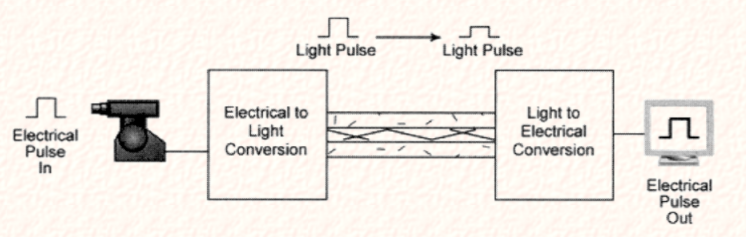top of page

# Transmission Lines- it's Parameters, Types, Properties and Applications

Updated: Aug 9

A #transmission line is a conducting structure designed and developed for the transmission of a large amount of electric power in the form of #electromagnetic waves from one station to another. They form a connection between the transmitter and receiver to allow the transmission of signals.

## Parameters of transmission lineThe above picture denotes the generalized equivalent circuit of a transmission line. The transmission line is characterized by four parameters. They are:

• Resistance: This parameter of the transmission line depends on the length and the area of the cross-section of the conducting material.

It is represented by R and its unit is ohms.

It is given by:Where;

ρ denotes the resistivity of the conducting material.

A denotes the cross-sectional area of the transmission line

L denotes the length of the transmission line.

• Capacitance: From the equivalent circuit diagram we can say that the transmission line is composed of two parallel conducting wires separated by a dielectric material. Hence, it has some #capacitance distributed uniformly over its length. It is represented by C and its unit is Farad.

• Inductance: When current flows through a transmission line, it generates a varying magnetic field perpendicular to the electric field. This varying magnetic field induces some emf in the transmission line that flows in the direction opposite to the current flow. This emf flowing in the opposite direction of current is called #inductance. It is denoted by L and its unit is Henry.

• Conductance: When current flows through the transmission line there is some kind of leakage current between the transmission line and ground. This leakage current leads to leakage #conductance in the transmission lines. It is denoted by G.

## Types of transmission lines

### a)Open wire transmission line:

It consists of two parallel conducting wires separated by a dielectric medium. Its one end is connected to the source and the other end is connected to the detector. It is one of the simplest forms of transmission line and costs low.### b)Coaxial transmission line:

It is formed when a conducting wire is inserted inside a hollow cylinder coaxially and the space between them is filled with dielectric materials such as air, gas, water, etc. This type of transmission line is used in CCTV systems, internet connections, television cables, etc.### C)Optic fiber transmission line:

It is used for the transmission of signals over long distances at the speed of light with the least dielectric losses. It works on the principle of total internal reflection. When light travels from the region of the higher refractive index to the lower refractive index it undergoes total internal reflection. This principle is used for the propagation of light in #opticalfiber. It has many advantages over coaxial cables. This type of transmission line is used in television cables, network connections, internet connections, etc.### d)Microstrip transmission line:

It is an unsymmetrical parallel plate line that has a dielectric substrate with a metalized ground at the bottom and a thin conducting strip on top with thickness 't' and width 'ω'.### e)Waveguides:

These types of transmission lines are used for the transmission of signals from one place to another at the #microwave frequency range. They are used in various devices for the measurement of optical and elastic properties of materials. There are two types of waveguides:

• Metallic waveguides: They are hollow conducting metal pipes used to carry high-frequency radio waves.• Dielectric waveguides: They consist of a dielectric material surrounded by another #dielectric material with a lower refractive index. They are used for the transmission of higher frequency radio waves.## Properties of transmission line:

### a)Characteristic impedance:

When an electromagnetic wave travels through a transmission line then the ratio of voltage across the transmission line and equivalent current flowing through it is called characteristic #impedance. The electromagnetic wave does not get reflected while traveling through the transmission line.

The general expression for characteristic impedance is given by:Where,

R represents the resistance per unit length

L represents the inductance per unit length

C represents the capacitance per unit length

G represents the conductance of the dielectric per unit length.

### b)Propagation constant:

It is the ratio of current measured at the output to the current applied at the input of the system.

The expression for #Propagationconstant is given by:γ=α+iβ

Where,

α is the attenuation constant

β is the phase constant

Io is the current measured at the output

Ii is the current applied at the input

## Applications of transmission lines

• Used for transmission of high-frequency signals over long as well as short distances.

• Used in television cables, internet connections, printed circuit boards, etc.

• Used for measuring optical and elastic properties of materials.

• Used in telephone lines.

• Used in voltage transformers